Schrodinger equation with particle in a box problem.

Three dimensional applications of the Schrodinger equation are introduced by the particle-in-a-box problem.

So far only a one-dimensional problem has been solved by application of the Schrodinger equation. Now the allowed energies and the probability functions for a particle that is free to move in three dimensions are deduced. A molecule of a gas enclosed in a cubic container provides a specific example that is dealt with in the section after the general procedure has been developed.

For any three-dimensional problem, the potential energy is, general, a function of three coordinates. For a cubic potential box, the Cartesian coordinates are convenient. The differential equation that must be solved is now the Schrodinger equation in three dimensions.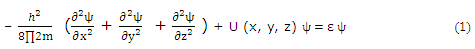For a "cubic box," the potential function can be expressed in terms of separate x, y, and z components,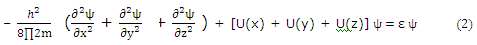Each of the potential function components for a "particle-in-a-box" is like the one-dimensional potential for a "particle-on-a-line".

For three-dimensional systems, the solution function ψ depends on the three coordinates necessary to locate a point in space. It is often profitable to try to separate such systems into parts, with each part involving only one coordinate. On the basis we try the substitution

ψ (x, y, z) = Ø(x)Ø(y)Ø(z)

Substitution of (2) from (1) gives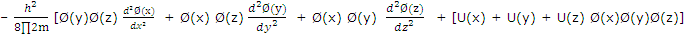Division by Ø(x)Ø(y)Ø(z) gives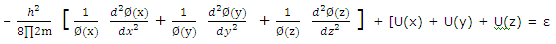For the equation to be satisfied for all values of x, y and z, each term on the left must equal a component of ε, and we can write

ε = εx + εy + εz

The Schrodinger equation can then be broken down into three identical equations of the type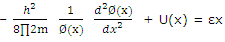Or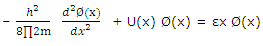These equations are identical to that written for one-dimensional problem. The solution to the three-dimensional cubic-box problem is therefore

ψ =  Ø(x)Ø(y)Ø(z)

With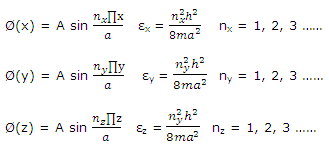#### Related Questions in Chemistry

• ##### Q :Mole fraction in vapours Choose the

Choose the right answer from following. If two substances A and B have P0A P0B= 1:2 and have mole fraction in solution 1 : 2 then mole fraction of A in vapours: (a) 0.33 (b) 0.25 (c) 0.52 (d) 0.2

• ##### Q :Linde liquefaction process Liquefied

Liquefied natural gas (LNG) is produced using a Linde liquefaction process from pure methane gas at 3 bar and 280 K (conditions at point 1 in figure below). A three-stage compressor with interceding is used to compress the methane to 100 bar (point 2). The first stage

• ##### Q :What are homogenous catalyst? Give few

When a catalyst mixes homogeneously with the reactants and forms a single phase, the catalyst is said to be homogeneous and this type of catalysis is called homogeneous catalysis. Some more examples of homogeneous catalysis are:    SO2

• ##### Q :Entropy is entropy on moleculare basis

is entropy on moleculare basis relates to the tras.,vib.,and rotational motions?

• ##### Q :Basic concept Give me answer of this

Give me answer of this question. The volume of water to be added to 100cm3 of 0.5 N N H2SO4 to get decinormal concentration is : (a) 400 cm3 (b) 500cm3 (c) 450cm3 (d)100cm3

• ##### Q :Non-ideal Gases Fugacity The fugacity

The fugacity is a pressure like quantity that is used to treat the free energy of nonideal gases.Now we begin the steps that allow us to relate free energy changes to the equilibrium constant of real, nonideal gases. The thermodynamic reaction

• ##### Q :Amines arrange in order of

arrange in order of basicity,pyridine,pipyridineand pyorine

• ##### Q :How molecule-molecule collisions takes

An extension of the kinetic molecular theory of gases recognizes that molecules have an appreciable size and deals with molecule-molecule collisions. We begin studies of elementary reactions by investigating the collisions b

• ##### Q :Solubility product On passing H 2 S gas

On passing H2S gas through a particular solution of Cu+ and Zn+2 ions, first CuS is precipitated because : (a)Solubility product of CuS is equal to the ionic product of ZnS (b) Solubility product of CuS is equal to the solubility product

• ##### Q :Negative deviation Which one of the

Which one of the following non-ideal solutions shows the negative deviation: (a) CH3COCH3 + CS2   (b) C6H6 + CH3COCH3   (c) CCl4 + CHCl3